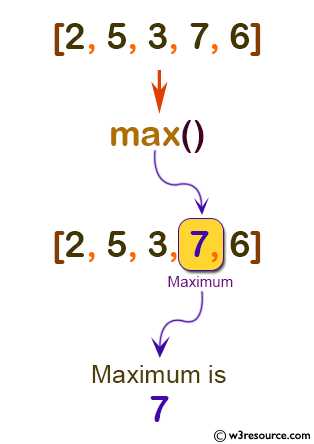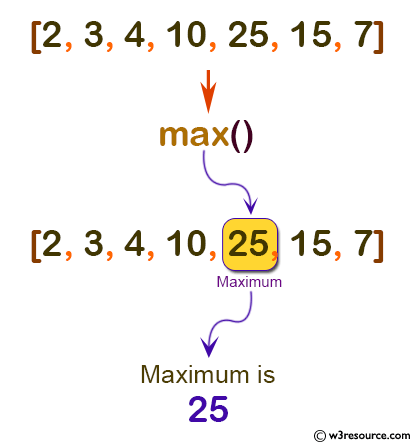# Python: max() function

## max() function

The max() function is used to find the item with the largest value in an iterable.

Version:

(Python 3.2.5)

Syntax:

```max(iterable, *[, key, default])

```

Parameter:

Name Description Required /
Optional
iterable An iterable, with one or more items to compare. Required
key Specifies a one-argument ordering function like that used for list.sort(). Optional
default Specifies an object to return if the provided iterable is empty. If the iterable is empty and default is not provided, a ValueError is raised. Optional

Syntax:

`max(arg1, arg2, *args[, key])`

Parameter:

Name Description Required /
Optional
arg1, arg2, ..args If one positional argument is provided, iterable must be a non-empty iterable. The largest item in the iterable is returned. If two or more positional arguments are provided, the largest of the positional arguments is returned. Required
key Specifies a one-argument ordering function like that used for list.sort(). Optional

Return value:

The largest item in the iterable is returned.

Example-1: Python max() function

``````# using max(arg1, arg2, *args)
print('Maximum is:', max(2, 5, 3, 7, 6))

# using max(iterable)
num = [2, 3, 4, 10, 25, 15, 7]
print('Maximum is:', max(num))
``````

Output:

```Maximum is: 7
Maximum is: 25
```

Pictorial Presentation:Pictorial Presentation:Example-2: Python max() function

``````x = [25, 100, 1500, 725]
y = [10, 5]
z = [35, 525, 98]

# using max(iterable, *iterables, key)
print('Maximum is:', max(x, y, z, key=len))
``````

Output:

```Maximum is: [25, 100, 1500, 725]
```

Python Code Editor:

Previous: map()
Next: memoryview()

Test your Python skills with w3resource's quiz

﻿

## Python: Tips of the Day

How do I check if a list is empty?

For example, if passed the following:

```a = []
if not a:
print("List is empty")
```

Ref: https://bit.ly/2A4JXx9